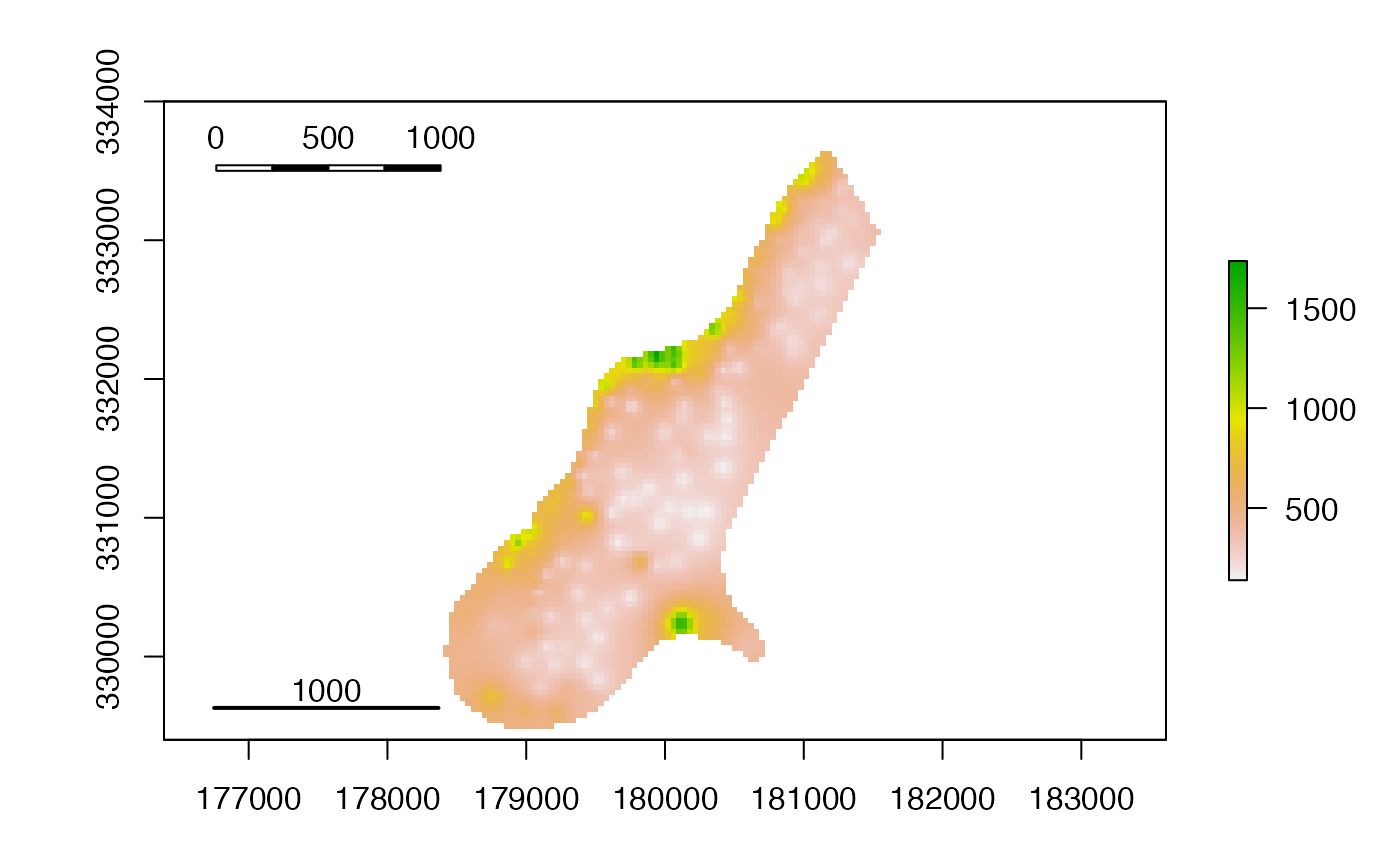Add a scalebar to a plot

scalebar(d, xy = NULL, type = "line", divs = 2, below = "",
lonlat = NULL, label, adj=c(0.5, -0.5), lwd = 2, ...)

## Arguments

d

distance covered by scalebar

xy

x and y coordinate to place the plot. Can be NULL. Use xy=click() to make this interactive

type

"line" or "bar"

divs

Number of divisions for a bar type. 2 or 4

below

Text to go below scalebar (e.g., "kilometers")

lonlat

Logical or NULL. If logical, TRUE indicates if the plot is using longitude/latitude coordinates. If NULL this is guessed from the plot's coordinates

label

Vector of three numbers to label the scale bar (beginning, midpoint, end)

lwd

line width for the "line" type scalebar

...

arguments to be passed to other methods

## Value

None. Use for side effect of a scalebar added to a plot

plot

## Author

Robert J. Hijmans; partly based on a function by Josh Gray

## Examples

f <- system.file("external/test.grd", package="raster")
r <- raster(f)
plot(r)
scalebar(1000)
scalebar(1000, xy=c(178000, 333500), type='bar', divs=4)